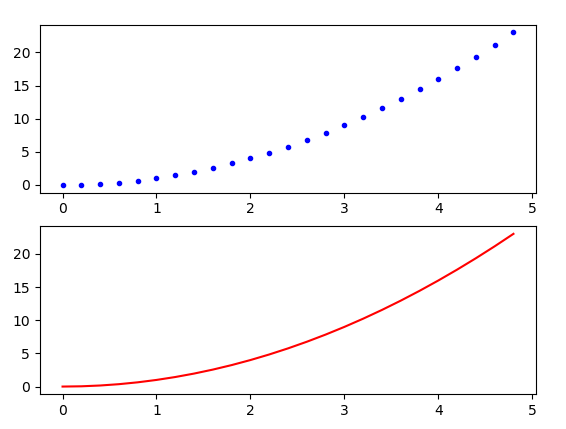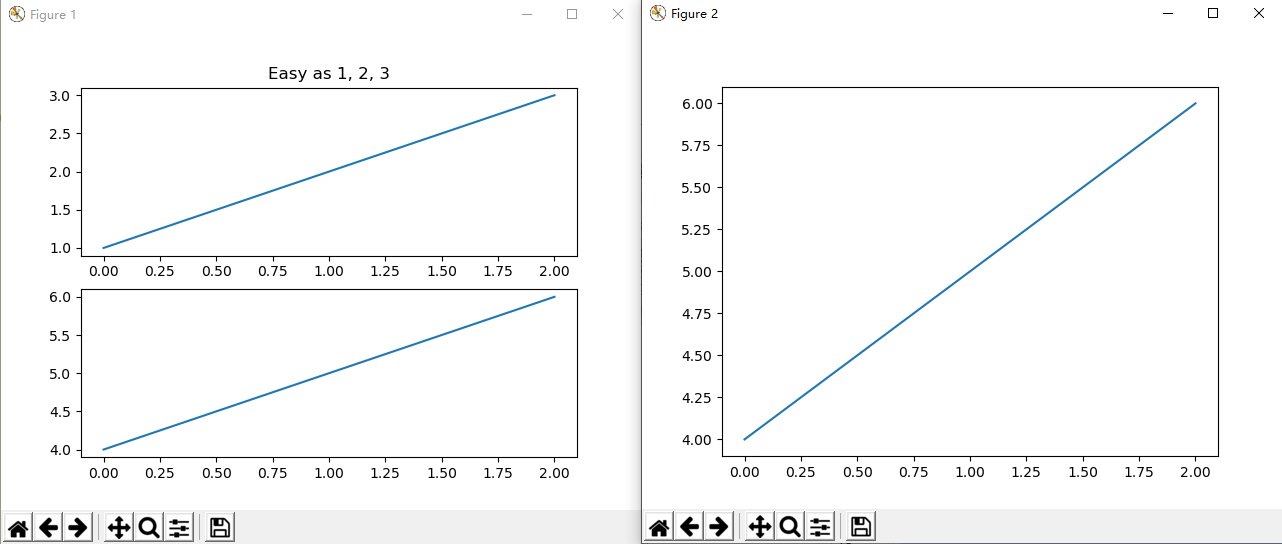# Matplotlib 绘图

Matplotlib 提供非常全面的数据可视化功能。

## 简单示例

import matplotlib.pyplot as plt

# 如果只传入一个数组作为参数， matplotlib 认为是 Y 轴的坐标
# 并自动产生 从 0 开始的 对应 X 轴坐标： 0、1、2、3 ...
plt.plot([2, 4, 6, 8])
plt.ylabel('some numbers')
plt.show()


import matplotlib.pyplot as plt

# 绘图点的 X 轴 坐标依次为 1, 3, 5, 7
# 绘图点的 Y 轴 坐标依次为 2, 4, 6, 8
plt.plot([1, 3, 5, 7], [2, 4, 6, 8])
plt.ylabel('some numbers')
plt.show()


import matplotlib.pyplot as plt

# 画一组数据
plt.plot([1, 2, 3, 4], [1, 4, 9, 16])
# 再画一组数据
plt.plot([1, 2, 3, 4], [1, 3, 5, 8])
plt.show()


## 显示中文字符

matplotlib的缺省字体不支持中文，我们可以指定一个支持中文的字体

import matplotlib.pyplot as plt

plt.rcParams['font.family'] = 'sans-serif'
# 设定字体为微软雅黑
plt.rcParams['font.sans-serif']=['Microsoft Yahei']

plt.plot([1, 2, 3, 4])
plt.xlabel('times 次数')
plt.show()


## 显示格式

b 表示 蓝色， - 表示 是线图。

import matplotlib.pyplot as plt

plt.plot([1, 2, 3, 4], [1, 4, 9, 16], 'r.')
plt.show()


## 指定宽度

import matplotlib.pyplot as plt

plt.plot(range(10), range(10), linewidth=0.5)
plt.show()


import matplotlib.pyplot as plt

plt.plot(range(10), range(10), 'r.', markersize=2.5)
plt.show()


## numpy 数组

import matplotlib.pyplot as plt
import numpy as np

# arange 就像 Python中的range
# 从 0 到 5 步长为 0.2
t = np.arange(0, 5, 0.2)

# 使用 numpy 的ndarray 作为数据
plt.plot(t, t**2, 'b.')
plt.show()


## 柱状图

import matplotlib.pyplot as plt

names = ['2016', '2017', '2018']
values = [1, 10, 100]

plt.bar(names, values)
plt.show()


## 多个子图（axes）

subplot 方法可以用来创建多个子图（axes）。

import matplotlib.pyplot as plt
import numpy as np

# arange 就像 Python中的range
# 从 0 到 5 步长为 0.2
t = np.arange(0, 5, 0.2)

# 指定2行，1列，共两个axe，当前使用第1个绘图块
plt.subplot(2,1,1)
plt.plot(t, t**2, 'b.')

# 当前使用第2个绘图块
plt.subplot(2,1,2)
plt.plot(t, t**2, 'r-')
plt.show()## 多个绘图（Figure）

matplotlib 每个绘图区都对应一个 Figure 对象。import matplotlib.pyplot as plt
plt.figure(1)                # the first figure
plt.subplot(211)             # the first subplot in the first figure
plt.plot([1, 2, 3])
plt.subplot(212)             # the second subplot in the first figure
plt.plot([4, 5, 6])

plt.figure(2)                # a second figure
plt.plot([4, 5, 6])          # creates a subplot(111) by default

plt.figure(1)                # figure 1 current; subplot(212) still current
plt.subplot(211)             # make subplot(211) in figure1 current
plt.title('Easy as 1, 2, 3') # subplot 211 title

plt.show()


## 图形中的文字

import matplotlib.pyplot as plt
import numpy as np

mu, sigma = 100, 15
x = mu + sigma * np.random.randn(10000)

n, bins, patches = plt.hist(x, 50, density=1, facecolor='g', alpha=0.75)

# x轴标题
plt.xlabel('Smarts')
# y轴标题
plt.ylabel('Probability')
# 子图标题
plt.title('Histogram of IQ')
# 指定坐标处添加文本
plt.text(60, .025, r'$\mu=100,\ \sigma=15$')
plt.axis([40, 160, 0, 0.03])
plt.grid(True)
plt.show()t = plt.xlabel('my data', fontsize=14, color='red')


VIP学员请联系老师获取补充练习